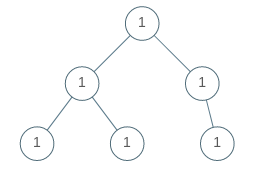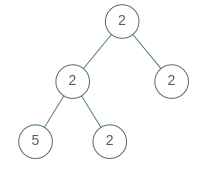# Question

Formatted question description: https://leetcode.ca/all/965.html

A binary tree is univalued if every node in the tree has the same value.

Return true if and only if the given tree is univalued.

Example 1:Input: [1,1,1,1,1,null,1]
Output: true
Example 2:Input: [2,2,2,5,2]
Output: false

Note:

The number of nodes in the given tree will be in the range [1, 100].
Each node's value will be an integer in the range [0, 99].



# Algorithm 1

This question defines a single-valued binary tree that requires all nodes in the binary tree to have the same value. First, I gave a binary tree and asked if it was a single-value binary tree. In fact, it is to investigate and traverse the binary tree, of course, the recursive method is the simplest in writing. Here, each node value can be compared with the root node value, as long as any one is different, it means that it is not a single-valued binary tree. Therefore, it is necessary to substitute the root node value as a parameter into the recursive function, so write a helper function to perform recursive writing of pre-order traversal, see the code as follows:

# Code 1

C++

class Solution {
public:
bool isUnivalTree(TreeNode* root) {
return helper(root, root->val);
}
bool helper(TreeNode* node, int val) {
if (!node) return true;
if (node->val != val) return false;
return helper(node->left, val) && helper(node->right, val);
}
};



# Algorithm 2

In a function comparison, as long as the values of the left and right child nodes of any node (if stored) are equal to the value of its parent node, it must be a single-valued binary tree. So in a function can also be compared, see the code as follows:

# Code 2

C++

class Solution {
public:
bool isUnivalTree(TreeNode* root) {
if (!root) return true;
if (root->left && root->left->val != root->val) return false;
if (root->right && root->right->val != root->val) return false;
return isUnivalTree(root->left) && isUnivalTree(root->right);
}
};



Java

• 
public class Univalued_Binary_Tree {

/**
* Definition for a binary tree node.
* public class TreeNode {
*     int val;
*     TreeNode left;
*     TreeNode right;
*     TreeNode(int x) { val = x; }
* }
*/
class Solution {

int uniValue;

public boolean isUnivalTree(TreeNode root) {

if (root == null) {
return true;
}

uniValue = root.val;

return dfs(root);
}

private boolean dfs (TreeNode root) {
if (root == null) {
return true;
}

return root.val == uniValue && dfs(root.left) && dfs(root.right);
}
}
}


# Algorithm 3

The above solutions are all recursive. Let’s look at the layer order traversal of iterative writing. There is no difference in the way of solving the problem, just the method of traversal is different. See the code as follows:

# Code 3

C++

class Solution {
public:
bool isUnivalTree(TreeNode* root) {
if (!root) return true;
queue<TreeNode*> q{ {root} };
while (!q.empty()) {
TreeNode* t = q.front(); q.pop();
if (t->val != root->val) return false;
if (t->left) q.push(t->left);
if (t->right) q.push(t->right);
}
return true;
}
};


• Todo

• # Definition for a binary tree node.
# class TreeNode(object):
#     def __init__(self, x):
#         self.val = x
#         self.left = None
#         self.right = None

class Solution(object):
def isUnivalTree(self, root):
"""
:type root: TreeNode
:rtype: bool
"""
q = collections.deque()
q.append(root)
val = root.val
while q:
node = q.popleft()
if not node:
continue
if val != node.val:
return False
q.append(node.left)
q.append(node.right)
return True